## 2.4Conservation of mass

The law of conservation of mass can be written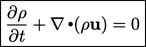(2.8)
where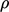is the mass density of the ﬂuid. The equation can be derived by considering a volume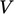ﬁxed in space (note !!), bounded by a surface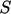. If the volume is ﬁlled by a ﬂuid with density, its mass is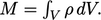The rate of increase of mass inside the volume must match the rate of inﬂow of mass across the volume’s surface. The latter is calculated by integrating the mass ﬂux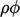over the surface, noting the negative sign due to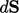pointing out of the volume.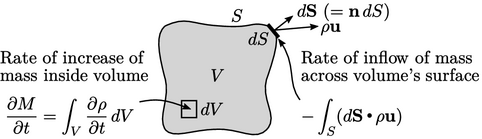Gauss’s Theorem relates surface and volume integrals by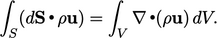(2.9)
Equating the rate of mass increase to rate of inﬂow and applying Eq. (2.9 ) gives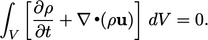(2.10)
Since the integral is valid for any volume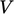, it follows that the integrand (in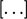) must equal 0, resulting in Eq. (2.8).

### Divergence

Divergence, denoted by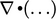, indicates the tendency of a vector ﬁeld to point outward of a closed surface. For example, When the divergence of velocity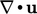is positive, the ﬂuid is expanding; negative divergence indicates contraction. Imagine a volume of ﬂuid with a complex distribution of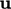at its bounding surface, below left. Thecalculation will isolate the diverging component (right) from uniform ﬂow (centre).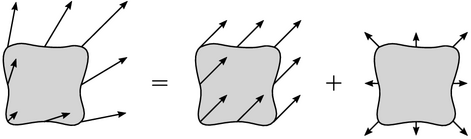The divergence of velocity is calculated by integrating — i.e. summing — ﬂuxes over the closed surface. To deﬁne divergence at a point, we consider the limiting case where the volume tends to zero. For a surface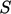that encloses a volume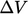, divergence is the ﬂux across the surface per unit volume, as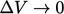, e.g.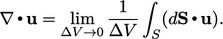(2.11)

### The nabla operator

The nabla symbol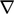can be considered a vector operator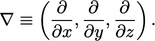(2.12)
Divergence is the inner product with, e.g.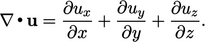(2.13)
Notes on CFD: General Principles - 2.4 Conservation of mass